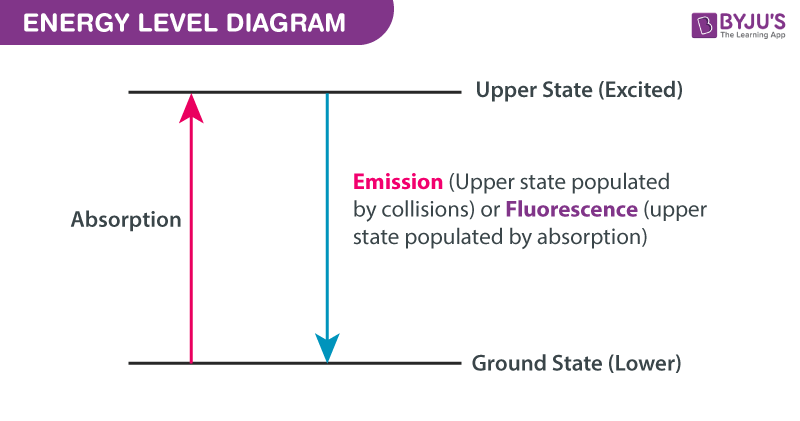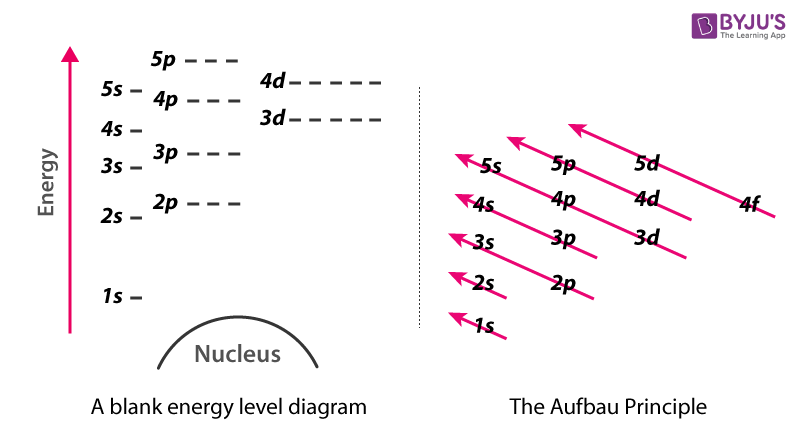# Energy Level Diagram

## What is energy level diagram?

In chemistry, an electron shell, or energy level, may be imagined as an orbit with electrons around the nucleus of an atom. The closest shell to the nucleus is called the “K shell” followed by the “L shell” then the “M shell” and so on away from the nucleus. The shells can be denoted by alphabets (K, L, M, …) or quantum numbers (n = 1, 2, 3, 4 and so on).

Each shell contains a fixed number of electrons –

• The first shell “K shell” contains two electrons,
• The second shell “L shell” contains eight electrons,
• The third shell “M shell” contains 18 electrons and so on.

The general formula to know the number of electrons that can be held in each shell is given by 2(n2). Electrons get attracted to the nucleus of the atom and the electrons occupy outer shells inner shells have already been completely occupied by other the electrons.

An atom or molecule or ion and its electrons are said to be in the ground state when they are at the lowest possible energy level. If they are at a higher energy level, they are said to be excited. Electrons that have higher energy than the ground state are said to be excited. They are then called degenerate energy levels occurs when more than one quantum mechanical state is at the same energy.Energy Level Diagram

## Understanding of energy level diagram:

With the help of the Grotrian diagram, we can understand that the light emission and absorption occur at the same wavelengths. This concept is named after a German astronomer Walter Grotrian, from the 20th century.

• When a molecule or atom absorbs light or colloids with another atom or ion which provides sufficient energy, it moves from a lower energy state to a higher energy state.
• Generally, the emission is initiated from an atom that was excited to its upper state either by collision or by absorption of surrounding light.

## How to represent electrons in an energy level diagram:

Chemists use an energy level diagram to seek information regarding the following:

• To anticipate what type of bonding will take place with a specific element and which electrons are being used.
• To demonstrate why definite elements behave in indistinguishable ways

Below is a blank energy level diagram which helps you depict electrons for any specific atom.At energy level 2, there are both s and p orbitals. The 2s has lower energy when compared to 2p. The three dashes in 2p subshells represent the same energy. 4s has lower energy when compared to 3d. Therefore, the order of energy level is as follows:

s<p<d<f

## Frequently Asked Questions on Energy Level Diagram

### What are the energy levels?

Electrons of an atom occupying particular orbitals have a particular energy. This is called energy level. When an electron alleviates from a high energy state to lower energy state emission of light occurs.

### What is an electron shell?

The space around the nucleus which is filled will force more electrons out from the nucleus. This is called an electron shell.

### What are the Lowest possible energy and Highest energy level of an electron?

The Lowest possible energy is ground state and Highest energy level is excited.

### Which ray has the highest energy level?

Gamma rays have the highest energy and part of it is harmful to human beings.

For more updates and latest notes on Energy level diagram and degenerate orbitals register to BYJU’S.

Test your knowledge on Energy Level Diagram